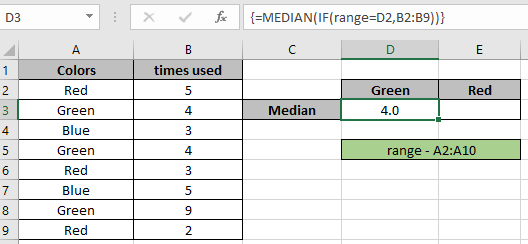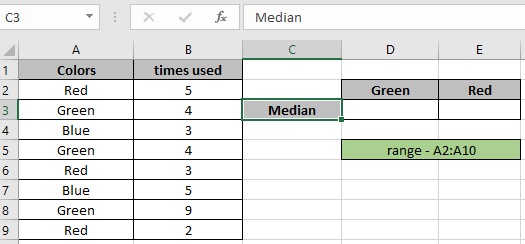# Calculate median with criteriaIn this article, we will learn how to calculate MEDIAN with criteria in Excel.
For instance, you have a large list of numbers in a data and you need to find the median of the numbers having a condition on it.

For this article we will be needing the use of the following functions:

1. IF Function
2. MEDIAN function

IF function is logic_test excel function. IF function tests a statement and returns values based on the result.

The MEDIAN function is a built-in Excel mathematical function. Median of a range of numbers is the middle value or middle number.

Median of values : 5,5,7 is 5 (middle number)
Median of values : 5,6,7,8 is 6.5 (average of the two medium values)

Now we will make a formula out of these functions to get median with criteria. Here we will be given the data and we need to find the median having a condition or criteria.

Generic formula:

{ = MEDIAN ( IF ( range = value , data )) }

Explanation:

• IF function checks the range with value and returns numbers from the data which matches TRUE with corresponding range.
• MEDIAN function takes only the numbers from data provided by the IF function criteria.

Note: Curly braces in excel must not be given manually instead use CTRL + SHIFT + ENTER to calculate arrays..

Let's test this formula via running it on an example:

### Example of Calculating Conditional Median

Here we have data having Colors and their time usage. First we need to find the median of the color Green.Now we will use the below formula to get MEDIAN.

Formula:

= MEDIAN ( IF ( range = D2 , B2:B9 ) )

Explanation:

• IF function checks the range with value "Green" in cell D2 and returns numbers from the data B2:B9 which matches TRUE with corresponding range.
• MEDIAN function takes only the numbers from data which are provided by the IF function criteria which are shown below.

= MEDIAN ( { FALSE ; 4 ; FALSE ; 4 ; FALSE ; FALSE ; 9 ; FALSE } )

• The MEDIAN function ignores FALSE values and returns MEDIAN for only the numbers.

Here the range is given as named reference & array to the function is given as cell_reference. Press Enter to get the result.As you can see in the above snapshot the MEDIAN of values extracted from the formula having criteria.

As you can see from the above formula the you can get conditional values.

Notes:

1. The MEDIAN function considers non - numeric values as 0s.
2. The MEDIAN function considers logic value TRUE as 1 and False as 0.
3. The argument array must be of same length else the function returns error.
4. The function returns 0 if the  Ctrl + Shift + Enter for curly braces is not used.

Hope this article about how to Return SUM only from formulas in Excel is explanatory. Find more articles on SUMPRODUCT functions here. Please share your query below in the comment box. We will assist you.

Related Articles

How to use the Median function in excel

How to use the IF function in excel

How to use the ISFORMULA function in Excel

How to Remove Text in Excel Starting From a Position

How to use the ROWS function in Excel

Validation of text entries

Create drop down list in excel with colour

Remove leading and trailing spaces from text in Excel

Popular Articles

50 Excel Shortcut to Increase Your Productivity

Edit a dropdown list

Absolute reference in Excel

If with conditional formatting

If with wildcards

Vlookup by date

Convert Inches To Feet and Inches in Excel 2016

Join first and last name in excel

Count cells which match either A or B

Terms and Conditions of use

The applications/code on this site are distributed as is and without warranties or liability. In no event shall the owner of the copyrights, or the authors of the applications/code be liable for any loss of profit, any problems or any damage resulting from the use or evaluation of the applications/code.